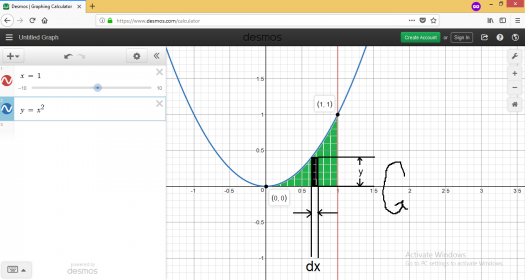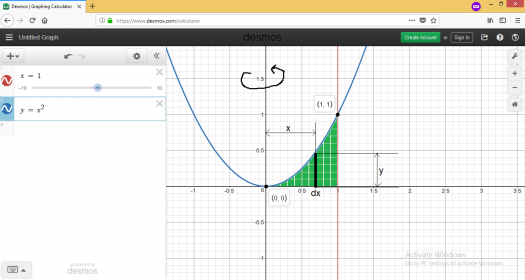# Integral calculus

Region 1 is bounded by the x axis, the line x=1 and the curve y=x^2.find the volume and centroid of the solid generated when the indicated region is revolved about y axis and revolved about x axis

Rate:### To get the volume and the

To get the volume and the centroid of the solid generated when the area bounded by the x-axis, line $x = 1$ and the curve $y = x^2$ is rotated about the x and y-axis, here it is:

To get the volume of the solid generated when the resulting area is rotated about x-axis, we need a figure. It looks like this:We can imagine that we could create a solid figure by using very small cylinders, so we will use the circular disk method to get the whole volume.The formula used for the circular disk method is...

$$V =\pi \int_a^b y^2 dx$$

Basing from the figure shown above, the equation we need is:

$$V = \pi \int_a^b y^2 dx$$

Then...

$$V = \pi \int_0^1 y^2 dx$$ $$V = \pi \int_0^1 (x^2)^2 dx$$ $$V = \pi \int_0^1 x^4 dx$$ $$V = \pi \int_0^1 x^4 dx$$ $$V = \frac{1}{5}\pi$$

The volume generated when the area is rotated around $x - axis$ is $\color{green}{\frac{1}{5}\pi \space cubic \space units}$

To get the centroid of the solid generated when the resulting area is rotated about x-axis, we will do this:

The general equation to get the centroid of a figure is...

$$V\bar x = \int x_c dV$$

where $V$ is the volume of the solid, $\bar x$ is the coordinate of the centroid of the resulting solid, and dV is the element of volume, usually a disk, ring or shell. Because of symmetry of the solid that lies on its axis, we only need one coordinate to find its centroid.

In this case, the equation we need to get the centroid is:

$$V\bar x = \int x_c dV$$

Now solving for the centroid:

$$V\bar x = \int x_c dV$$ $$\left( \frac{1}{5} \pi\right)\bar x = \int_0^1 x (\pi y^2 dx)$$ $$\left( \frac{1}{5} \pi\right)\bar x = \int_0^1 x (\pi (x^2)^2 dx)$$ $$\left( \frac{1}{5} \pi\right)\bar x = \int_0^1 \pi x^5 dx$$ $$\left( \frac{1}{5} \pi\right)\bar x = \frac{1}{6} \pi$$ $$x_c = \frac{5}{6}$$

Therefore, the centroid of the volume that lies on x - axis is $\color{green}{x_c = \frac{5}{6}}$

To get the volume of the solid generated when the resulting area is rotated about y-axis, we need a figure. It looks like this:We can imagine that we could create a solid figure by using very small cylindrical shells, so we will use the cylindrical shell method to get the whole volume.
The formula used for the circular disk method is...

$$V = \int_a^b 2\pi x y dx$$

The volume of the cylindrical shell is expressed as circumference of circle $\times$ height $\times$ thickness or...
The volume of the cylindrical shell is expressed as $2\pi r$ $\times$ $h$ $\times$ $t$ or in this problem's context...
The volume of the cylindrical shell element $dV$ is expressed as $2\pi x$ $\times$ $y$ $\times$ $dx$.

Basing from the figure shown above, the equation we need is:

$$V = \int_a^b 2\pi x y^2 dx$$

Then solving for its volume:

$$V = \int_a^b 2\pi x y dx$$ $$V = \int_0^1 2\pi x (x^2) dx$$ $$V = \int_0^1 2\pi x (x^4) dx$$ $$V = \int_0^1 2\pi x^5 dx$$ $$V = \frac{1}{2}\pi$$

The volume generated when the area is rotated around y - axis is $\color{green}{\frac{1}{2}\pi \space cubic \space units}$

To get the centroid of the solid generated when the resulting area is rotated about y-axis, we will do this:

The general equation to get the centroid of a figure is...

$$V\bar x = \int x_c dV$$

where $V$ is the volume of the solid, $\bar x$ is the coordinate of the centroid of the resulting solid, and dV is the element of volume, usually a disk, ring or shell. Because of symmetry of the solid that lies on its axis, we only need one coordinate to find its centroid.

In this case, the equation we need to get the centroid is:

$$V\bar y = \int y_c dV$$

Now solving for the centroid:

$$V\bar y = \int y_c dV$$ $$\left( \frac{1}{2} \pi\right)\bar y = \int_0^1 \frac{1}{2}y (2\pi xy dx)$$ $$\left( \frac{1}{2} \pi\right)\bar y = \int_0^1 y \pi xy dx$$ $$\left( \frac{1}{2} \pi\right)\bar y = \int_0^1 \pi xy^2 dx$$ $$\left( \frac{1}{2} \pi\right)\bar y = \int_0^1 \pi x(x^2)^2 dx$$ $$\left( \frac{1}{2} \pi\right)\bar y = \int_0^1 \pi x^5 dx$$ $$\left( \frac{1}{2} \pi\right)\bar y = \frac{1}{6} \pi$$ $$y_c = \frac{1}{3}$$

Therefore, the centroid of the volume that lies on y - axis is $\color{green}{y_c = \frac{1}{3}}$

Sana makakatulong...:-)

Rate: